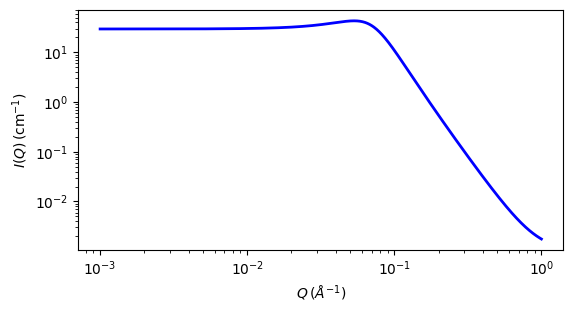# teubner_strey

Teubner-Strey model of microemulsions

Parameter

Description

Units

Default value

scale

Scale factor or Volume fraction

None

1

background

Source background

cm-1

0.001

volfraction_a

Volume fraction of phase a

None

0.5

sld_a

SLD of phase a

10-6-2

0.3

sld_b

SLD of phase b

10-6-2

6.3

d

Domain size (periodicity)

100

xi

Correlation length

30

The returned value is scaled to units of cm-1 sr-1, absolute scale.

Definition

This model calculates the scattered intensity of a two-component system using the Teubner-Strey model. Unlike dab this function generates a peak. A two-phase material can be characterised by two length scales - a correlation length and a domain size (periodicity).

The original paper by Teubner and Strey defined the function as:

$I(q) \propto \frac{1}{a_2 + c_1 q^2 + c_2 q^4} + \text{background}$

where the parameters $$a_2$$, $$c_1$$ and $$c_2$$ are defined in terms of the periodicity, $$d$$, and correlation length $$\xi$$ as:

$\begin{split}a_2 &= \biggl[1+\bigl(\frac{2\pi\xi}{d}\bigr)^2\biggr]^2\\ c_1 &= -2\xi^2\bigl(\frac{2\pi\xi}{d}\bigr)^2+2\xi^2\\ c_2 &= \xi^4\end{split}$

and thus, the periodicity, $$d$$ is given by

$d = 2\pi\left[\frac12\left(\frac{a_2}{c_2}\right)^{1/2} - \frac14\frac{c_1}{c_2}\right]^{-1/2}$

and the correlation length, $$\xi$$, is given by

$\xi = \left[\frac12\left(\frac{a_2}{c_2}\right)^{1/2} + \frac14\frac{c_1}{c_2}\right]^{-1/2}$

Here the model is parameterised in terms of $$d$$ and $$\xi$$ and with an explicit volume fraction for one phase, $$\phi_a$$, and contrast, $$\delta\rho^2 = (\rho_a - \rho_b)^2$$ :

$I(q) = \frac{8\pi\phi_a(1-\phi_a)(\Delta\rho)^2c_2/\xi} {a_2 + c_1q^2 + c_2q^4}$

where $$8\pi\phi_a(1-\phi_a)(\Delta\rho)^2c_2/\xi$$ is the constant of proportionality from the first equation above.

In the case of a microemulsion, $$a_2 > 0$$, $$c_1 < 0$$, and $$c_2 >0$$.

For 2D data, scattering intensity is calculated in the same way as 1D, where the $$q$$ vector is defined as

$q = \sqrt{q_x^2 + q_y^2}$Fig. 122 1D plot corresponding to the default parameters of the model.

Source

teubner_strey.py

References

1. M Teubner, R Strey, J. Chem. Phys., 87 (1987) 3195

2. K V Schubert, R Strey, S R Kline and E W Kaler, J. Chem. Phys., 101 (1994) 5343

3. H Endo, M Mihailescu, M. Monkenbusch, J Allgaier, G Gompper, D Richter, B Jakobs, T Sottmann, R Strey, and I Grillo, J. Chem. Phys., 115 (2001), 580

Authorship and Verification

• Author: